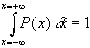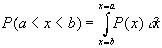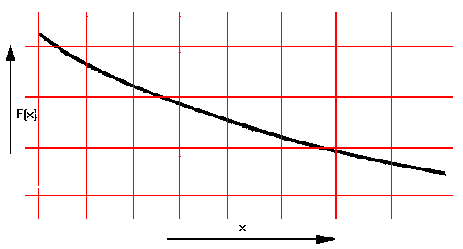# Maths - Statistics - Continous Probability Distributions

P(x) = probability density function.ie total area under graph = certancy = 1ie probability of x being between a and b= Expected value of x = ( average)= Varience of x = ( standard deviation)

## Normal Distribution

Often applies where sample size is large.0.3 Probability density = P(x)

=

0.2

0.1

## PoissonDistributionprobability of r events in time t

= average no of

events in time t

mean =

varience =

## Exponential DistributionE(x) =

V(x) =